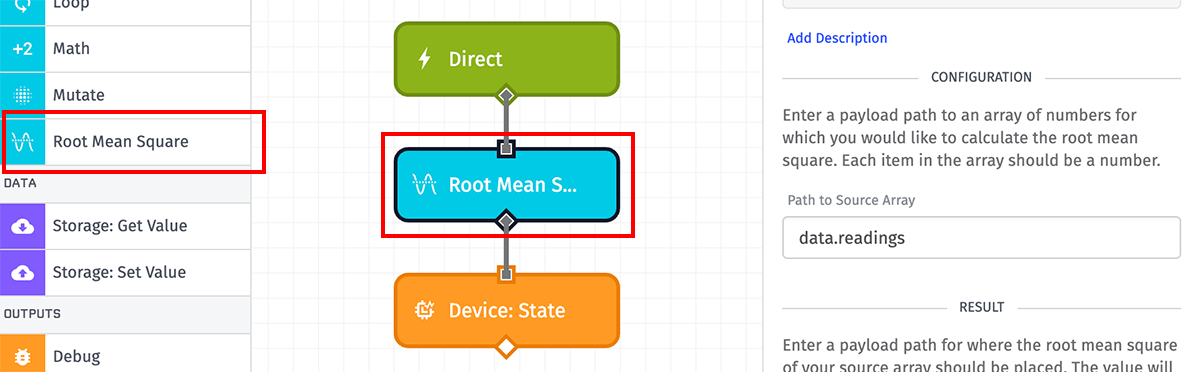# Root Mean Square Node

The Root Mean Square Node allows for calculating the root mean square (RMS) of an array of numbers and placing the calculated value at a payload path of your choosing.## Configuration

There are two properties to set for the Root Mean Square Node.

### Path to Source Array

First, enter a payload path to an array of numbers for which you would like to calculate the root mean square. Each entry in the array must be a number or the node will throw an error.

Note: The maximum length of the source array is 2,048 items. If your source array has more items than that limit, the node will error.

### Result Path

Next, enter a payload path for where the result of the node should be placed on your payload. The value placed at this path will be the calculated root mean square, or an `error` object if the RMS could not be calculated.

## Node Errors

There are a handful of common cases where the Root Mean Square Node will throw an error:

• If the value at the source path is not an array.
• If the value is an array but the array is empty.
• If any item within the source array is not a number.
• If the length of the source array is greater than 2,048 items.